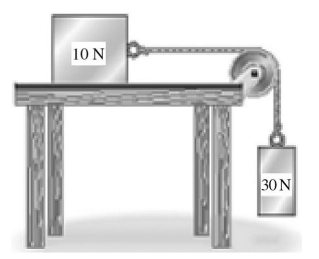# Problem: Two boxes are connected to each other by a string as shown in the figure. The 10-N box slides without friction on the horizontal table surface. The pulley is ideal and the string has negligible mass. What is true about the tension T in the string?A) T = 30 NB) T &lt; 30 NC) T = 10 ND) T &gt; 30 NE) T = 20 N

###### FREE Expert Solution

This problem involves forces. We'll use Newton's second law to solve for the unknown force

Newton's second law:

$\overline{){\mathbf{\Sigma }}{\mathbf{F}}{\mathbf{=}}{\mathbf{m}}{\mathbf{a}}}$

94% (317 ratings)###### Problem Details

Two boxes are connected to each other by a string as shown in the figure. The 10-N box slides without friction on the horizontal table surface. The pulley is ideal and the string has negligible mass. What is true about the tension T in the string?A) T = 30 N
B) T < 30 N
C) T = 10 N
D) T > 30 N
E) T = 20 N